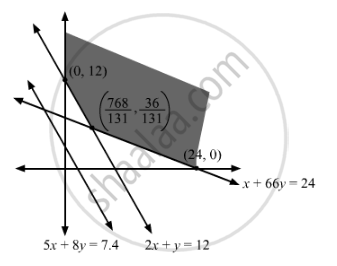# A Firm Manufactures Headache Pills in Two Sizes a and B.Determine Also the Quantity of Codeine Consumed by Patient. - Mathematics

Sum

A firm manufactures headache pills in two sizes A and B. Size A contains 2 grains of aspirin, 5 grains of bicarbonate and 1 grain of codeine; size B contains 1 grain of aspirin, 8 grains of bicarbonate and 66 grains of codeine. It has been found by users that it requires at least 12 grains of aspirin, 7.4 grains of bicarbonate and 24 grains of codeine for providing immediate effects. Determine graphically the least number of pills a patient should have to get immediate relief. Determine also the quantity of codeine consumed by patient.

#### Solution

Let the number of size A pill be and the number of size B pill be y.
Therefore, the constraints are
$\begin{array}{lc}2x + y \geq 12 & \\ 5x + 8y \geq 7 . 4 & \\ x + 66y \geq 24 &\end{array}$

z = x + y  which is to be minimisedThe corner points are (0, 12), (24, 0) and $\left( \frac{768}{131}, \frac{36}{131} \right)$
The values of Z at these corner points are as follows

 Corner point Z = x + y (0, 12) 12 (24, 0) 24 $\left( \frac{768}{131}, \frac{36}{131} \right)$ 6.1373

The minimum value of Z is 6.1373 but the region is unbounded so check whether x + y < 6.1373 has common region with the feasible solution.
Clearly, it can be seen that it doesn't has any common region.

So,
$x = \frac{768}{131}, y = \frac{36}{131}$
This is the least quantity of pill A and B.
$\begin{array}{l}\text{ Codline quantity } \Rightarrow \frac{768}{131} + 66 \times \frac{36}{131} \\ \Rightarrow 24 \hspace{0.167em} \text{ grains }| \end{array}$
Concept: Graphical Method of Solving Linear Programming Problems
Is there an error in this question or solution?

#### APPEARS IN

RD Sharma Class 12 Maths
Chapter 30 Linear programming
Exercise 30.4 | Q 16 | Page 52